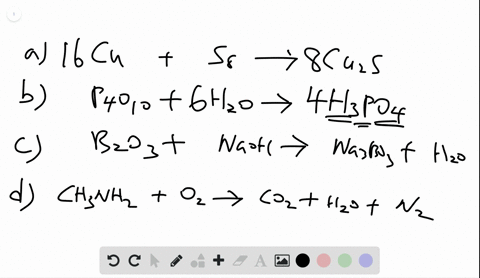🎉 The Study-to-Win Winning Ticket number has been announced! Go to your Tickets dashboard to see if you won! 🎉View Winning Ticket### Write balanced equations for each of the followin…

05:51University of Maine
Problem 55

# The scenes below represent a chemical reaction between elements A (red) and B (green):Which best represents the balanced equation for the reaction?$\begin{array}{ll}{\text { (a) } 2 \mathrm{A}+2 \mathrm{B} \longrightarrow \mathrm{A}_{2}+\mathrm{B}_{2}} & {\text { (b) } \mathrm{A}_{2}+\mathrm{B}_{2} \longrightarrow 2 \mathrm{AB}} \\ {\text { (c) } \mathrm{B}_{2}+2 \mathrm{AB} \longrightarrow 2 \mathrm{B}_{2}+\mathrm{A}_{2}} & {\text { (d) } 4 \mathrm{A}_{2}+4 \mathrm{B}_{2} \longrightarrow 8 \mathrm{AB}}\end{array}$

## Discussion

You must be signed in to discuss.

## Video Transcript

given a visual representation of the molecules in a chemical reaction, we can write a balanced chemical equation. In this image, you can see that there are two different types of reactive A, which is red and B, which is green. They're connected as molecules, so we need to consider each one as a die atomic molecule, which we write as a to plus B two. So each one of the two circles together represents a molecule, and you can see that there are 1234 molecules of a to and there are 1234 molecules of B two. On the product side, the A and the B have broken apart to make a new molecule that has one A and one B, So we call it a B. There are a total of 12345678 of these. So the chemical equation is for a two plus four. B two makes eight a B, and this is balanced because on this side we look at the number of A's. There are four times two or eight, and on this side, there also ate bees on the side. If we look at how many A's and B's there are The eight multiplies both so there eight days and eight bees. So this is the balanced equation.